Power in a Series CircuitCustom SearchVoltage in a Series CircuitMatter, Energy, and ElectricityRules for Series DC CircuitsPower in a Series Circuit

Each of the resistors in a series circuit consumes power which is dissipated in the form of heat. Since this power must come from the source, the total power must be equal to the power consumed by the circuit resistances. In a series circuit the total power is equal to the SUM of the power dissipated by the individual resistors. Total power (PT) is equal to:

PT = P1 + P 2 + P3 . . .Pn

Example: A series circuit consists of three resistors having values of 5 ohms, 10 ohms, and 15 ohms. Find the total power when 120 volts is applied to the circuit. (See fig. 3-19.)

Figure 3-19. - Solving for total power in a series circuit.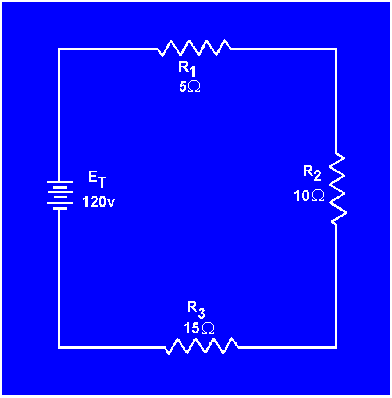Given: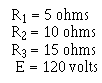Solution: The total resistance is found first.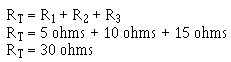By using the total resistance and the applied voltage, the circuit current is calculated.By means of the power formulas, the power can be calculated for each resistor: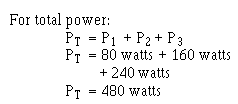To check the answer, the total power delivered by the source can be calculated: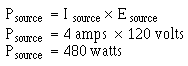The total power is equal to the sum of the power used by the individual resistors.

SUMMARY OF CHARACTERISTICS
The important factors governing the operation of a series circuit are listed below. These factors have been set up as a group of rules so that they may be easily studied. These rules must be completely understood before the study of more advanced circuit theory is undertaken.Integrated Publishing, Inc. - A (SDVOSB) Service Disabled Veteran Owned Small Business